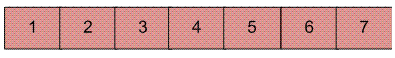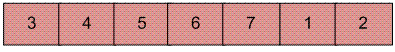# Reversal algorithm for array rotation

Write a function rotate(arr[], d, n) that rotates arr[] of size n by d elements.
Example :

```Input :  arr[] = [1, 2, 3, 4, 5, 6, 7]
d = 2
Output : arr[] = [3, 4, 5, 6, 7, 1, 2]

```Rotation of the above array by 2 will make arrayThe first 3 methods to rotate an array by d elements has been discussed in this post.
Method 4 (The Reversal Algorithm) :
Algorithm :

```rotate(arr[], d, n)
reverse(arr[], 1, d) ;
reverse(arr[], d + 1, n);
reverse(arr[], 1, n);

```

Let AB are the two parts of the input array where A = arr[0..d-1] and B = arr[d..n-1]. The idea of the algorithm is :

• Reverse A to get ArB, where Ar is reverse of A.
• Reverse B to get ArBr, where Br is reverse of B.
• Reverse all to get (ArBr) r = BA.

Example :
Let the array be arr[] = [1, 2, 3, 4, 5, 6, 7], d =2 and n = 7
A = [1, 2] and B = [3, 4, 5, 6, 7]

• Reverse A, we get ArB = [2, 1, 3, 4, 5, 6, 7]
• Reverse B, we get ArBr = [2, 1, 7, 6, 5, 4, 3]
• Reverse all, we get (ArBr)r = [3, 4, 5, 6, 7, 1, 2]

Below is the implementation of the above approach :

## C++

 `// C++ program for reversal algorithm` `// of array rotation` `#include ` `using` `namespace` `std;`   `/*Function to reverse arr[] from index start to end*/` `void` `reverseArray(``int` `arr[], ``int` `start, ``int` `end)` `{` `    ``while` `(start < end) {` `        ``int` `temp = arr[start];` `        ``arr[start] = arr[end];` `        ``arr[end] = temp;` `        ``start++;` `        ``end--;` `    ``}` `}`   `/* Function to left rotate arr[] of size n by d */` `void` `leftRotate(``int` `arr[], ``int` `d, ``int` `n)` `{` `    ``if` `(d == 0)` `        ``return``;` `    ``// in case the rotating factor is` `    ``// greater than array length` `    ``d = d % n;`   `    ``reverseArray(arr, 0, d - 1);` `    ``reverseArray(arr, d, n - 1);` `    ``reverseArray(arr, 0, n - 1);` `}`   `// Function to print an array` `void` `printArray(``int` `arr[], ``int` `size)` `{` `    ``for` `(``int` `i = 0; i < size; i++)` `        ``cout << arr[i] << ``" "``;` `}`   `/* Driver program to test above functions */` `int` `main()` `{` `    ``int` `arr[] = { 1, 2, 3, 4, 5, 6, 7 };` `    ``int` `n = ``sizeof``(arr) / ``sizeof``(arr);` `    ``int` `d = 2;`   `    ``// Function calling` `    ``leftRotate(arr, d, n);` `    ``printArray(arr, n);`   `    ``return` `0;` `}`

## C

 `// C/C++ program for reversal algorithm of array rotation` `#include `   `/*Utility function to print an array */` `void` `printArray(``int` `arr[], ``int` `size);`   `/* Utility function to reverse arr[] from start to end */` `void` `reverseArray(``int` `arr[], ``int` `start, ``int` `end);`   `/* Function to left rotate arr[] of size n by d */` `void` `leftRotate(``int` `arr[], ``int` `d, ``int` `n)` `{`   `    ``if` `(d == 0)` `        ``return``;` `    ``// in case the rotating factor is` `    ``// greater than array length` `    ``d = d % n;`   `    ``reverseArray(arr, 0, d - 1);` `    ``reverseArray(arr, d, n - 1);` `    ``reverseArray(arr, 0, n - 1);` `}`   `/*UTILITY FUNCTIONS*/` `/* function to print an array */` `void` `printArray(``int` `arr[], ``int` `size)` `{` `    ``int` `i;` `    ``for` `(i = 0; i < size; i++)` `        ``printf``(``"%d "``, arr[i]);` `}`   `/*Function to reverse arr[] from index start to end*/` `void` `reverseArray(``int` `arr[], ``int` `start, ``int` `end)` `{` `    ``int` `temp;` `    ``while` `(start < end) {` `        ``temp = arr[start];` `        ``arr[start] = arr[end];` `        ``arr[end] = temp;` `        ``start++;` `        ``end--;` `    ``}` `}`   `/* Driver program to test above functions */` `int` `main()` `{` `    ``int` `arr[] = { 1, 2, 3, 4, 5, 6, 7 };` `    ``int` `n = ``sizeof``(arr) / ``sizeof``(arr);` `    ``int` `d = 2;`   `    ``leftRotate(arr, d, n);` `    ``printArray(arr, n);` `    ``return` `0;` `}`

## Java

 `// Java program for reversal algorithm of array rotation` `import` `java.io.*;`   `class` `LeftRotate {` `    ``/* Function to left rotate arr[] of size n by d */` `    ``static` `void` `leftRotate(``int` `arr[], ``int` `d)` `    ``{`   `        ``if` `(d == ``0``)` `            ``return``;`   `        ``int` `n = arr.length;` `        ``// in case the rotating factor is` `        ``// greater than array length` `        ``d = d % n;` `        ``reverseArray(arr, ``0``, d - ``1``);` `        ``reverseArray(arr, d, n - ``1``);` `        ``reverseArray(arr, ``0``, n - ``1``);` `    ``}`   `    ``/*Function to reverse arr[] from index start to end*/` `    ``static` `void` `reverseArray(``int` `arr[], ``int` `start, ``int` `end)` `    ``{` `        ``int` `temp;` `        ``while` `(start < end) {` `            ``temp = arr[start];` `            ``arr[start] = arr[end];` `            ``arr[end] = temp;` `            ``start++;` `            ``end--;` `        ``}` `    ``}`   `    ``/*UTILITY FUNCTIONS*/` `    ``/* function to print an array */` `    ``static` `void` `printArray(``int` `arr[])` `    ``{` `        ``for` `(``int` `i = ``0``; i < arr.length; i++)` `            ``System.out.print(arr[i] + ``" "``);` `    ``}`   `    ``/* Driver program to test above functions */` `    ``public` `static` `void` `main(String[] args)` `    ``{` `        ``int` `arr[] = { ``1``, ``2``, ``3``, ``4``, ``5``, ``6``, ``7` `};` `        ``int` `n = arr.length;` `        ``int` `d = ``2``;`   `        ``leftRotate(arr, d); ``// Rotate array by d` `        ``printArray(arr);` `    ``}` `}` `/*This code is contributed by Devesh Agrawal*/`

## Python

 `# Python program for reversal algorithm of array rotation`   `# Function to reverse arr[] from index start to end`     `def` `reverseArray(arr, start, end):` `    ``while` `(start < end):` `        ``temp ``=` `arr[start]` `        ``arr[start] ``=` `arr[end]` `        ``arr[end] ``=` `temp` `        ``start ``+``=` `1` `        ``end ``=` `end``-``1`   `# Function to left rotate arr[] of size n by d`     `def` `leftRotate(arr, d):`   `    ``if` `d ``=``=` `0``:` `        ``return` `    ``n ``=` `len``(arr)` `    ``# in case the rotating factor is` `    ``# greater than array length` `    ``d ``=` `d ``%` `n` `    ``reverseArray(arr, ``0``, d``-``1``)` `    ``reverseArray(arr, d, n``-``1``)` `    ``reverseArray(arr, ``0``, n``-``1``)`   `# Function to print an array`     `def` `printArray(arr):` `    ``for` `i ``in` `range``(``0``, ``len``(arr)):` `        ``print` `arr[i],`     `# Driver function to test above functions` `arr ``=` `[``1``, ``2``, ``3``, ``4``, ``5``, ``6``, ``7``]` `n ``=` `len``(arr)` `d ``=` `2`   `leftRotate(arr, d)  ``# Rotate array by 2` `printArray(arr)`   `# This code is contributed by Devesh Agrawal`

## C#

 `// C# program for reversal algorithm` `// of array rotation` `using` `System;`   `class` `GFG {` `    ``/* Function to left rotate arr[]` `    ``of size n by d */` `    ``static` `void` `leftRotate(``int``[] arr, ``int` `d)` `    ``{`   `        ``if` `(d == 0)` `            ``return``;` `        ``int` `n = arr.Length;` `          ``// in case the rotating factor is` `        ``// greater than array length` `        ``d = d % n;` `        ``reverseArray(arr, 0, d - 1);` `        ``reverseArray(arr, d, n - 1);` `        ``reverseArray(arr, 0, n - 1);` `    ``}`   `    ``/* Function to reverse arr[] from` `    ``index start to end*/` `    ``static` `void` `reverseArray(``int``[] arr, ``int` `start,` `                             ``int` `end)` `    ``{` `        ``int` `temp;` `        ``while` `(start < end) {` `            ``temp = arr[start];` `            ``arr[start] = arr[end];` `            ``arr[end] = temp;` `            ``start++;` `            ``end--;` `        ``}` `    ``}`   `    ``/*UTILITY FUNCTIONS*/` `    ``/* function to print an array */` `    ``static` `void` `printArray(``int``[] arr)` `    ``{` `        ``for` `(``int` `i = 0; i < arr.Length; i++)` `            ``Console.Write(arr[i] + ``" "``);` `    ``}`   `    ``// Driver code` `    ``public` `static` `void` `Main()` `    ``{` `        ``int``[] arr = { 1, 2, 3, 4, 5, 6, 7 };` `        ``int` `n = arr.Length;` `        ``int` `d = 2;`   `        ``leftRotate(arr, d); ``// Rotate array by 2` `        ``printArray(arr);` `    ``}` `}`   `// This code is contributed by Sam007`

## PHP

 ``

Output :

```3 4 5 6 7 1 2

```

Time Complexity : O(n)

Attention reader! Don’t stop learning now. Get hold of all the important DSA concepts with the DSA Self Paced Course at a student-friendly price and become industry ready.

My Personal Notes arrow_drop_up

Article Tags :
Practice Tags :

196

Please write to us at contribute@geeksforgeeks.org to report any issue with the above content.